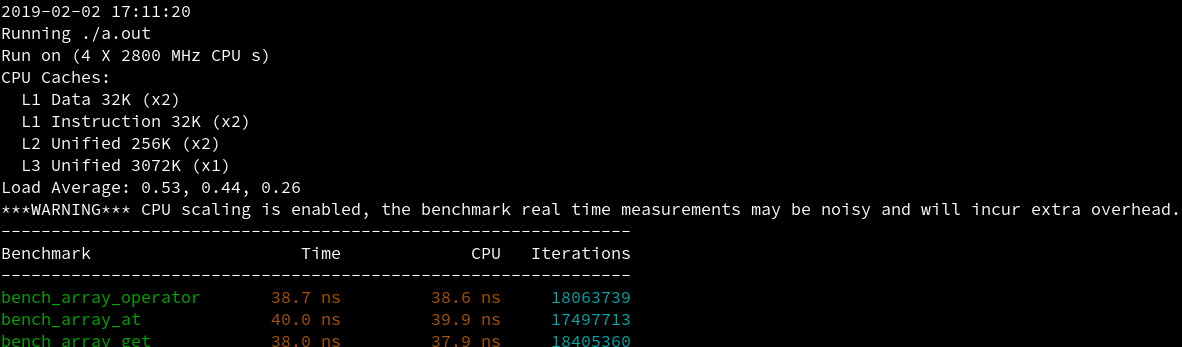Debian/Ubuntu:

sudo apt install g++ cmake


Arch Linux/Manjaro Linux:

sudo pacman -s g++ cmake


git clone https://github.com/google/benchmark.git
mkdir build && cd build
cmake -DCMAKE_BUILD_TYPE=RELEASE ../benchmark
make -j4
# 如果想全局安装就接着运行下面的命令
sudo make install


#include <benchmark/benchmark.h>
#include <array>

constexpr int len = 6;

// constexpr function具有inline属性，你应该把它放在头文件中
constexpr auto my_pow(const int i)
{
return i * i;
}

// 使用operator[]读取元素，依次存入1-6的平方
static void bench_array_operator(benchmark::State& state)
{
std::array<int, len> arr;
constexpr int i = 1;
for (auto _: state) {
arr = my_pow(i);
arr = my_pow(i+1);
arr = my_pow(i+2);
arr = my_pow(i+3);
arr = my_pow(i+4);
arr = my_pow(i+5);
}
}
BENCHMARK(bench_array_operator);

// 使用at()读取元素，依次存入1-6的平方
static void bench_array_at(benchmark::State& state)
{
std::array<int, len> arr;
constexpr int i = 1;
for (auto _: state) {
arr.at(0) = my_pow(i);
arr.at(1) = my_pow(i+1);
arr.at(2) = my_pow(i+2);
arr.at(3) = my_pow(i+3);
arr.at(4) = my_pow(i+4);
arr.at(5) = my_pow(i+5);
}
}
BENCHMARK(bench_array_at);

// std::get<>(array)是一个constexpr function，它会返回容器内元素的引用，并在编译期检查数组的索引是否正确
static void bench_array_get(benchmark::State& state)
{
std::array<int, len> arr;
constexpr int i = 1;
for (auto _: state) {
std::get<0>(arr) = my_pow(i);
std::get<1>(arr) = my_pow(i+1);
std::get<2>(arr) = my_pow(i+2);
std::get<3>(arr) = my_pow(i+3);
std::get<4>(arr) = my_pow(i+4);
std::get<5>(arr) = my_pow(i+5);
}
}
BENCHMARK(bench_array_get);

BENCHMARK_MAIN();


g++ -Wall -std=c++14 benchmark_example.cpp -pthread -lbenchmark


benchmark需要链接libbenchmark.so，所以需要指定-lbenchmark，此外还需要thread的支持，因为libstdc++不提供thread的底层实现，我们需要pthread。另外不建议使用-lpthread，官方表示会出现兼容问题，在我这测试也会出现链接错误。注意文件名一定要在-lbenchmark前面，否则编译会失败，具体参见：https://github.com/google/benchmark/issues/619posted @ 2019-02-02 18:11  apocelipes  阅读(10919)  评论(1编辑  收藏  举报